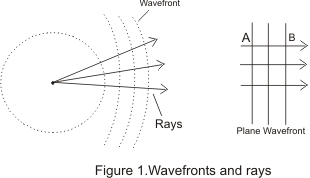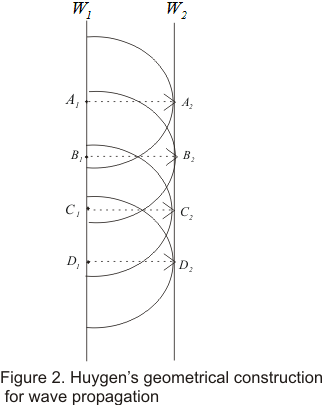# Huyghen's principle and interference of light

### 1. Introduction

• Light is a form of energy .This fact was predicted by Maxwell on theoretical grounds and was verified by Lebedew experimentally in 1901
• Since light is a form of energy, its transmission from one place to another can be understood in terms of transmission of energy
• There are only two modes of propagation of energy through any material medium
1. energy is carried by stream of material particles travelling with finite velocity
2. Transfer of energy by wave motion without actual travelling with matter
• First mode of energy transfer leads to Newton�s corpuscular theory of light in which he tried to understand travel of light in the straight line assuming that luminous body emits very minute and weightless particles called corpuscles travelling through empty space in straight lines in all directions with the speed of light and carry KE with them
• This corpuscular theory of light can fairly explain the phenomenon of reflection .refraction and rectilinear propagation of light but failed to explain the phenomenon of interference, diffraction and polarization etc
• Second mode of energy transfer leads the wave theory of light which was put forward by Dutch physicist Christian Huygens in 1678
• Huygens suggested that light may be a wave phenomenon produced by mechanical vibrations of an all pervading hypothetical homogenous medium called eather just like those in solids and liguid .This medium was supposed to be mass less with extremely high elasticity and very low density
• At first wave theory of light was not accepted primarily because of Newton�s authority and also light could travel through vacuum and waves require a medium to propagate from one point to other
• Wave theory of light first begin to gain acceptance when double slit experiment of Thomas Young in 1801 established that light is indeed a wave phenomenon
• After Young's double slit interference experiment ,many experiments were carried out by scientists involving interference and diffraction of light waves which could only be satisfactory explained by assuming wave model of light
• Later on in nineteenth century Maxwell put forwards his electromagnetic theory and predicted the existence of electromagnetic waves and calculated the speed of EM waves in free space and found that this value was very close to the measured value of speed of light
• He then suggested light must be an EM wave associated with changing electric and magnetic field which result in the propagation of light or EM waves in vacuum .So no material medium ( like ether suggested by Huygens) is required for the propagation of light wave from one place to another .This argument established that light is a wave phenomenon
• In this chapter we will study the various phenomenon related to wave nature of light

## 2) Wave fronts and rays

• Consider the figure given below in which a point source of light S starts a distance or wave in air• These waves will travel in all directions with the same velocity c, which is the velocity of light
• After time t, distance travelled by the wave would be equal to ct and light energy thus reaches the surface of the sphere of radius ct with S as its center as shown in figure 1
• The surface of such a sphere is known as wave front of light at this instant and all the particles forming wave front are in the same phase of vibration
• With the passage of time wave travels farther and new wave fronts are obtained .These are all the surfaces of spheres of center S
• Thus at any instant of time wave front may be defined as the locus of all the particles in the medium which are being distributed at the same instant of time and are in the same phase of vibration
• At points very far away from source S such as A or B the wave fronts are parts of the sphere of very large radius so at any such large distances from source wave fronts are substantially plane
• Rays are defined as normal�s to the wave fronts and in case of plane wave fronts ,rays are all parallel to one another as shown in figure 1.

## 3) Huygens�s principle

• Huygens principle of wave propagation is a geometrical description used to determine the new position of a wave front at later time from its given position at any given instant of time. It is based on two principles
1. Each point on a given or primary wave front acts like a new source sending out disturbance in all directions and are known as secondary wavelets
2. The envelope or the tangential plane to these secondary wavelets consitutes the new wave front
• To understand the propagation of wave on the basis of these postulates consider the figure given below• For simplicity we are considering the simple case of a plane wave
a) Let at time t=0,W1 be the wave front which spates those part of the medium which are undisturbed from those where wave has already reached
b) Each point on W1 acts as a source of secondary waves by sending out spherical wave of radius vt where v is the velocity of the wave
c) After some time t, the disturbance in the medium reaches all points within the region covered by all t these secondary waves. The boundary of this region is new wave front W2 and W2 is the surface tangent to all spheres and is known as forward envelope of these secondary wave fronts
d) The secondary wave fro pint A1 on W1 touches W2 at A2.The line connecting point A1 and A2 on wavelength W1 and W2 respectively is a ray of length vt.This is the reason why rays are perpendicular to the wave fronts
e) We can repeat this construction starting with W2 to get the next wave fronts W3 at some later time t2 and so on
• We have explained Huygens construction using a place wave fronts but the construction is more general than our simple example .The wave fronts can have any shape and sped of waves can be different at different places and in different direction's